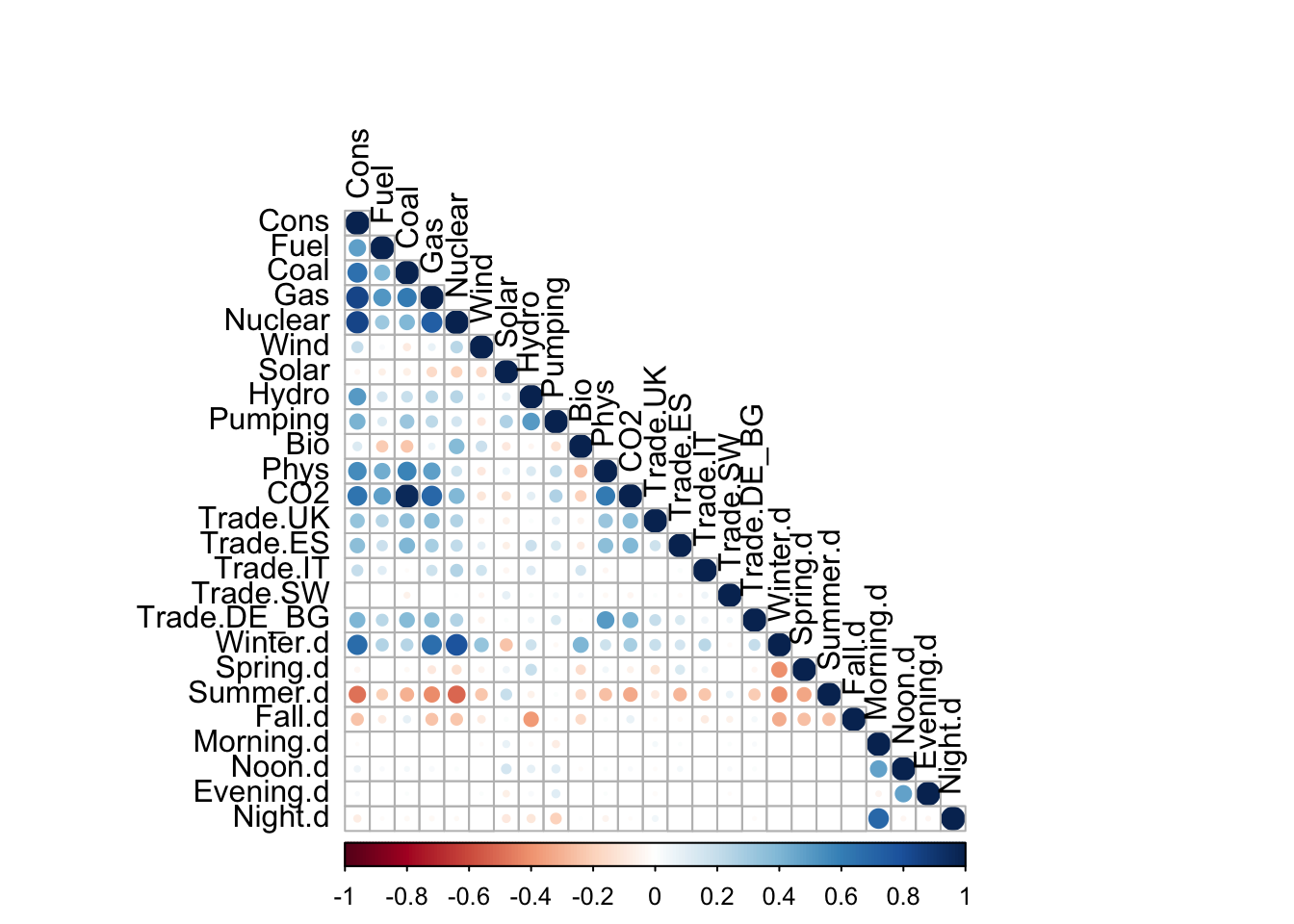### Introduction

We have performed analysis of electricity data provided by the French Distribution Network (RTE)

### Analysis

1. Background on electricity in France
2. Correlation studies
3. Consumption patterns
4. Imports and export
5. Evolution of the energy mix

Source

### Background on electricity in France

The file has the following structure. It has 52608 rows and 23 columns.
The maximum consumption at one point in time in France between 2012 and 2014 was 102098 MW. This is located on row 1864 and happened on 2/8/2012. This is lower than the electrical available power which amounts to 129 310 MW as of 12/31/15.

### Correlation studies

The purpose of the current analysis is to interpret French Energy System data. The raw data contains the following information about French Energy System for every 30 minute during the period from 1/1/2012 untill 12/31/2014:

1. Total consumption of energy (demand) in MW (megawatts);
2. Generation mix that meets the demand. The generators are grouped according to the nature of energy producing units and fuel used;
3. Net export data that describes energy flows of French Energy System and energy systems of other companies
4. CO2 emission data recorded aggregately for all energy generators.

Given the nature of the data the analysis will focuse on the following issues: 1. Understanding of correlations between consumption, generation mix, CO2 emissions, net export, time of the day and season. 2. If correlation is traced and can be explained the next step is to use linear regression models to describe the relationship between dependent and independent variables.

#### Correlation matrix analysis

We used R programming language tools to construct correlation matrix for the parameters selected from the raw dataset. For better visualisation we used “Corrplot” package, that can let us trace dependencies in a convenient way. We decided to exclude from analysis the correlation coefficients that are not significant under p = 0,05. Such cells are left blank in the following diagramm:The exact correlation coefficients betwenn selected parameters are given in the followng matrix:

As we can see from the table and diagramm above there are correlations between following variables:

1. Consumption and generation mix;
2. Consumption and the season of the year;
3. CO2 emissions and fuel-fired generators (primarily Coal and Gas);
4. Solar energy generation and the season;
5. Wind energy generation and the season.

The correlation between consumption and generation mix seems obvious since power stations produce electricity according to the current demand load in the system. We decided not to construct the model for this relationship since similar analysis is provided in the energy mix table. Instead, we decided to futher investigate relationships from point 2 to 5.

#### Regression of consumption vc the season of the year

To find the relationship between consumption of the energy and the season we assumed that Intercept will represent consumption in Fall and used other seasons are either 1 or 0. The results of regression are the following:

$$Consumption = 4.7916\times 10^{4} + -3457*Summer +6055*Spring +1.8877\times 10^{4}*Winter$$

#### Regression of CO2 emissions vc fuel-fired generators (primarily Coal and Gas)

To understand relationship between CO2 emissions and energy generated from different sources (generation mix) we ran the following regression:

$$CO2_emission = 0.0076902*Fuel + 0.0148562*Coal +5.651\times 10^{-4}*Gas +4.885\times 10^{-4}*Nuclear$$ summary(Regression.CO2)

#### Regression of Solar energy generation vc the season

To find the relationship between solar energy generation and the season we assumed that Intercept will represent generation in Fall and used other seasons are either 1 or 0. The results of regression are the following:

$$Solar_generation = 571 + 235*Summer +46*Spring +-315*Winter$$

#### Regression of Wind energy generation vc the season

To find the relationship between wind energy generation and the season we assumed that Intercept will represent generation in Fall and used other seasons are either 1 or 0. The results of regression are the following:

$$Wind_generation = 1555 + -277*Summer +163*Spring +891*Winter$$

### Consumption patterns

#### Daily consumption in winter and summer

The chart below shows energy needs in summer and winter. It compares the instant energy consumption on Jan 1, 2012 and Jul 1, 2012. The two curves share the same pattern however in winter, the power need is generally higher because of heating. Besides, summer in France is not very hot so there are not many air-conditioners installed which normally consumer a lot of electricity.